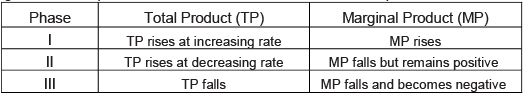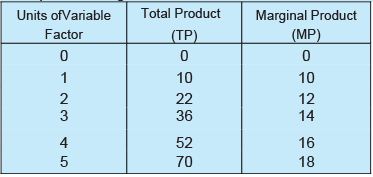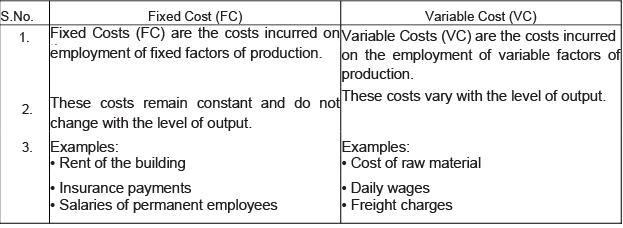Courses

# Short Questions With Answers - Production And Costs Commerce Notes | EduRev

## Commerce : Short Questions With Answers - Production And Costs Commerce Notes | EduRev

The document Short Questions With Answers - Production And Costs Commerce Notes | EduRev is a part of the Commerce Course Economics Class 11.
All you need of Commerce at this link: Commerce

Q.1. Define Marginal Physical Product.
Ans.
Marginal Physical Product (MPP) or Marginal Product (MP) is the change in the Total Physical Product due to a unit increase in the employment of a variable input.

Q.2. What is meant by Total Physical Product?
Ans.
Total Physical Product (TPP) or Total Product (TP) is the total output produced by a firm using its variable inputs during a period of time..

Q.3. How is Total Product derived from the Marginal Product schedule?
Ans.
Total Product is derived by summing the Marginal Product of each unit of variable input.

Q.4. What will you say about the Marginal Product of a factor when Total Product is falling?
Ans.
When Total Product is falling the Marginal Product will be negative.

Q.5. What is the general shape of the MP curve?
Ans.
MP curve is an inverted U-shaped curve (n).

Q.6. What is the general shape of the AP curve?
Ans.
AP curve is an inverted U-shaped curve (n).

Q.7. Explain the likely behaviour of Total Product and Marginal Product when only one input is increased while all other inputs are kept unchanged.
Ans.
The Law of Variable Proportions explains the behaviour of Total Product and Marginal Product. It states that when more and more units of a variable factor are employed, keeping the other factors fixed, the Total Product (TP) first increases at an increasing rate, then increases at a diminishing rate and finally starts falling. The different phases in the behaviour of TP and MP can be explained as below.Q.8. What do ‘returns to scale’ refer to?
Ans.
Returns to scale studies the change in output in the long run due to a change in the scale of production. All the factors of production are variable and there is no fixed factor.

Q.9. List any three inputs used in production.
Ans.
Inputs used in production include:
(i) Land
(ii) Labour
(iii) Capital

Q.10. What is the Law of Variable Proportions?
Ans.
Law of Variable Proportions states that as more and more units of the variable factor are employed, other inputs remaining constant, the Total Product (TP) first increases at an increasing rate, then increases at a diminishing rate and finally starts falling.

Q.11. Define returns to a factor.
Ans.
Returns to a factor refer to the effects of changes in variable factor of production on output, when other factors remain fixed.

Q.12. Give meaning of ‘returns to scale’.
Ans.
Returns to scale studies the change in output in the long-run due to a change in the scale of production. All the factors of production are variable and there is no fixed factor.

Ans.
A production function satisfies decreasing returns to scale when a percentage change in output is less than the percentage change in all inputs.

Q.14. Give the meaning of increasing returns to scale.
Ans.
When the proportional increase in all inputs results in an increase in output by more than the proportion, it is called increasing returns to scale.

Q.15. Give the meaning of constant returns to scale.
Ans.
When the proportional increase in all inputs results in an increase in output by the same proportion, it is called constant returns to scale.

Q.16. What is meant by returns to a factor? What leads to increasing returns to factor? Explain.

Ans. Returns to a factor means the increase in the Total Product (TP) per unit increase in the employment of a variable factor, when the employment of other factors is kept constant.
Increasing Marginal Product leads to increasing returns to factor. In other words, when Total Product increases at an increasing rate, increasing returns to factor takes place. This can be explained with the help of following table.In the table, Marginal Product increases by employing an additional unit of variable factor. That is, Total Product is increasing at an increasing rate. Thus, increasing MP leads to increasing returns of factor.

Q.17. What is meant by decreasing returns to factor? Give causes of its application.
Ans.
Decreasing returns to factor implies that keeping other inputs constant, an increase in the variable input will cause the Marginal Product to decrease after a certain level of output. It is also known as the Law of Diminishing Marginal Returns.
Following are the causes of Diminishing Marginal Returns to Factor:
(i) Fixity of Factors: When more and more units of variable factor are combined with the fixed factor, the latter gets over-utilised. As a result, the Marginal Product from each additional unit of variable factor declines.
(ii) Imperfect Substitution: Factors of production are imperfect substitutes of each other. It is possible to use one factor as a substitute for another factor of production only till a limit. However, production beyond that limit causes diminishing marginal returns.
(iii) Scarcity of Factors of Production: Every factor of production is available in limited quantity. Availability of limited factor decreases as the level of output is increases. Consequently, the cost increases and marginal product starts to decline.

Q.18. What is Fixed Costs?
Ans.
Fixed Costs (FC) are the costs incurred on the employment of fixed factors of production. These costs remain fixed at all the levels of output.

Q.19. Define Variable Costs.
Ans.
Variable Costs (VC) are incurred on the employment of variable factors of production. These costs costs which vary with the level of output.

Q.20. What does cost mean in economics?
Ans.
Costs refer to the total expenditure incurred by a firm on the production of a good. It is the sum of actual and imputed expenditure on the inputs by the owner.

Q.21. Give meaning of ‘opportunity cost’.
Ans.
Opportunity cost refers to the cost of next best alternative foregone while making a choice or decision.

Q.22. What is meant by real cost?
Ans.
The overall cost of producing a commodity is called real cost.

Q.23. Why is Average Total Cost greater than Average Variable Cost?
Ans.
Average Total Cost (ATC) is greater than Average Variable Cost because it is the sum of Average Variable Cost (AVC) and Average Fixed Cost (AFC). That is, AC - AFC + AVC

Q.24. How does Average Fixed Cost behave as output is increased?
Ans.
Average Fixed Cost (AFC) goes on declining as the level of output increases because Fixed Cost is constant at every level of output. However, it never becomes zero.

Q.25. Give two examples of Fixed Costs.
Ans.
Examples of Fixed Costs include:
(i) Rent
(ii) Wages of permanent employees

Q.26. Give two examples of Variable Costs.
Ans.
Examples of Variable Costs include:
(i) Purchase of raw material
(ii) Wages of casual labour

Q.27. Distinguish between Fixed Costs and Variable Costs. Give two examples of each.
Ans. Following are the points of difference between Fixed Cost and Variable Cost:Offer running on EduRev: Apply code STAYHOME200 to get INR 200 off on our premium plan EduRev Infinity!

,

,

,

,

,

,

,

,

,

,

,

,

,

,

,

,

,

,

,

,

,

;